# Integration - Calculus Notes | Study Engineering Mathematics - GATE

## GATE: Integration - Calculus Notes | Study Engineering Mathematics - GATE

The document Integration - Calculus Notes | Study Engineering Mathematics - GATE is a part of the GATE Course Engineering Mathematics.
All you need of GATE at this link: GATE

Introduction

Let us consider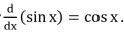We observe that the cos x is the derivative function of sin x or we can say that sin x is an anti-derivative (or an integral) of cos x.

Some Important Integrals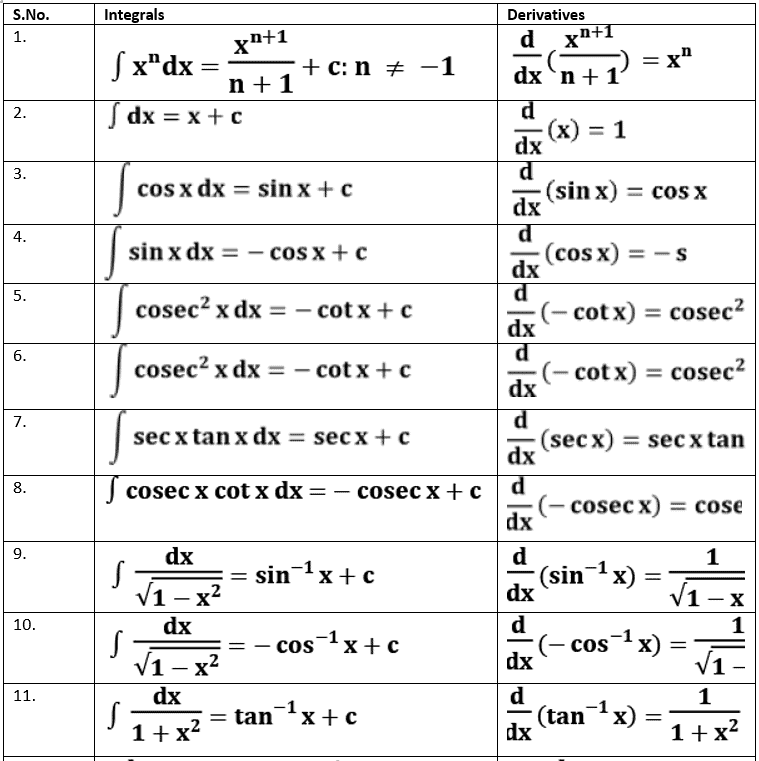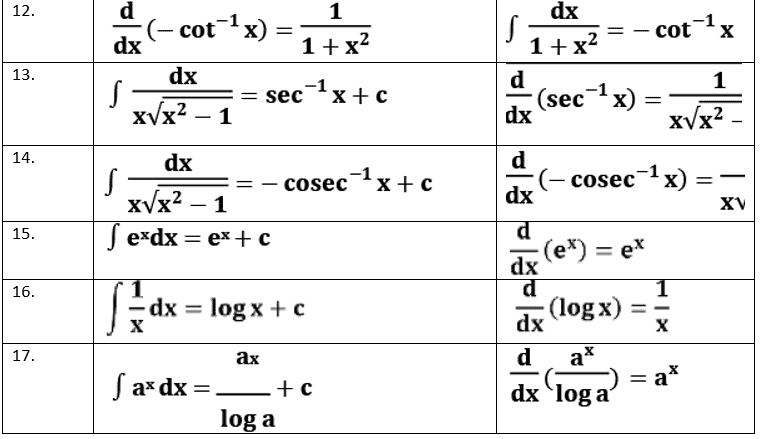Some Properties of Indefinite Integrals

1. ∫ [f(x) + g(x)] dx = ∫f(x)dx + ∫g(x)dx
2. ∫kf(x)dx = k∫f(x)dx where k is constant
3. ∫f'(ax + b)dx =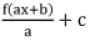4. ∫sinh x dx = cosh x + c
5. ∫cosh x dx = sinh x + c
6. ∫tanh x dx = log |cosh x| + c
7. ∫coth x dx = log |sinh x| + c
8. ∫sech x dx = tan-1 |sinh x | + c
9. ∫cosec2h x dx = –coth x + c
10. ∫cosech x  coth x = –cosech x + c
11. ∫sech x  tanh x dx = –sech x + c

Methods of Integration

• Integration by substitution
• Integration using Trigonometric Identities
• Integration using partial fractions
• Integration by parts

1. Integration by Substitution
The given integral ∫f(x)dx can be transformed into another form by changing the independent variable x to t by substituting x = g(t)   Consider, I = ∫f(x)dx
Put, x = g(t) so that dx / dt = g'(t)
dx = g'(t)dt
I = ∫f(x)dx = ∫f(g(t)) g'(t)dt

Example 1:
Integrate the following function,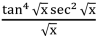Solution:
Derivative of √x is = 1 / 2√x
We use the substitution √x  = t
So, that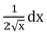= dt ⇒ dx = 2t dt
Thus,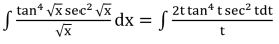= 2∫tan4 t sec2 t dt
Again we make another substitution tan t = u
sec2 t dt = dx
= 2∫ x4 dx = 2u5/ 5 + c
=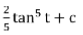[since u = tan t]
=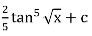Note: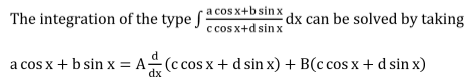2. Integration using Trigonometric Identities
The following example can be solved by using trigonometric Identities

Example 2:
∫cos2x dx
Solution:
We know,  cos 2x = 2 cos2 x – 1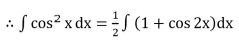=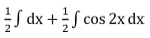=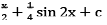Integrals of Some Particular Functions

1.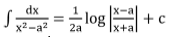2.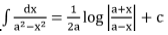3.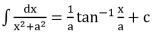4.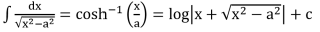5.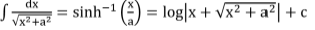To find the integral of type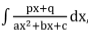, where p,q,a,b,c are constants we need to find real numbers A, B such that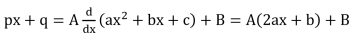To determine A and B we equate from both sides the coefficients of x and the constant terms.

3. Integration by Partial Fractions
The following example can be solved by using partial fraction method

Example 3:
Find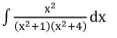Solution: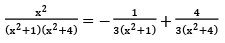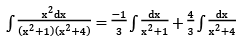=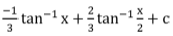4. Integration by Parts
This can be done by using the given formula
f(x)g(x)dx = f(x)∫g(x)dx – ∫[∫g(x)dx] f'(x)dx
In integration by parts method proper choice of first and second function:
The first function is the word ILATE
I → Inverse Trigonometric
L → Logarithmic
A → Algebraic
T → Trigonometric
E → Exponential

Example 4:
Find the value of  ∫x cos x dx
Solution:
Put f(x) = x (first function) and g(x) = cos x (second function)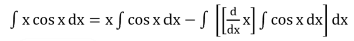= x sin x – ∫sin x dx
= x sin x + cos x + c

Integrals of Some Particular Functions

1.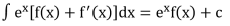2.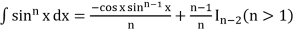3.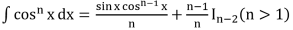4.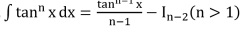5.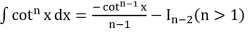6.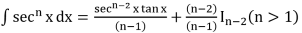7.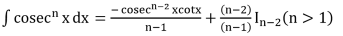8.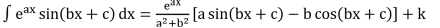9.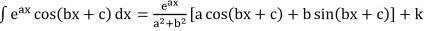10.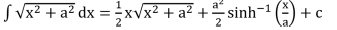11.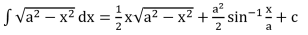Definite Integrals

A real valued function f(x) is continuous on [a, b] then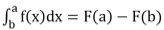is called definite integral. Geometrically it gives area of finite regions.

Properties of Definite Integrals

1.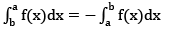2.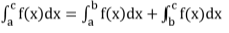3.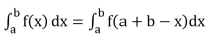4.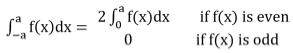5.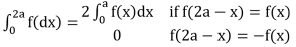6.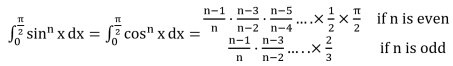7.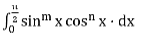=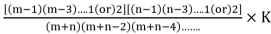where k = π/2 ; if m and n are even
= 1 ; otherwise

Example 5:
Find the value of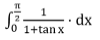Solution:
Let, I =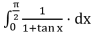Also, I =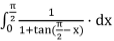=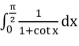=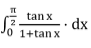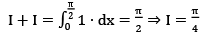Solving Indefinite Integrals Using Functions
There are two functions which can be used to solve improper indefinite integrals. They are given as

A. Gamma Function
Gamma Function is defined by: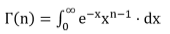Properties

1. Γ(1) = 1
2. Γ(2) = 1
3. Γ(3) = 2
4. Γ(n+1) = nΓ(n) = n!
5.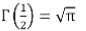6.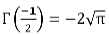7.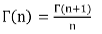B. β – Function (Beta Function)
It is defined as β(m, n) =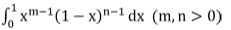Properties

1. β(m, n) = β(n, m)
2. β(m, n) =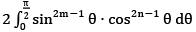3. β(m, n) =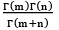4.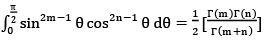Example 6:
Find the value of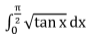Solution: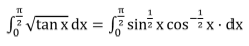2m - 1 = 1 / 2
⇒ m = 3 / 4
⇒ 2n - 1 = -1 / 2
⇒ n = 1 / 4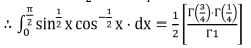The document Integration - Calculus Notes | Study Engineering Mathematics - GATE is a part of the GATE Course Engineering Mathematics.
All you need of GATE at this link: GATEUse Code STAYHOME200 and get INR 200 additional OFF

## Engineering Mathematics

55 docs|21 tests

Track your progress, build streaks, highlight & save important lessons and more!

,

,

,

,

,

,

,

,

,

,

,

,

,

,

,

,

,

,

,

,

,

;